2017-01-20

## " Voronoi Bike Concept (2016) "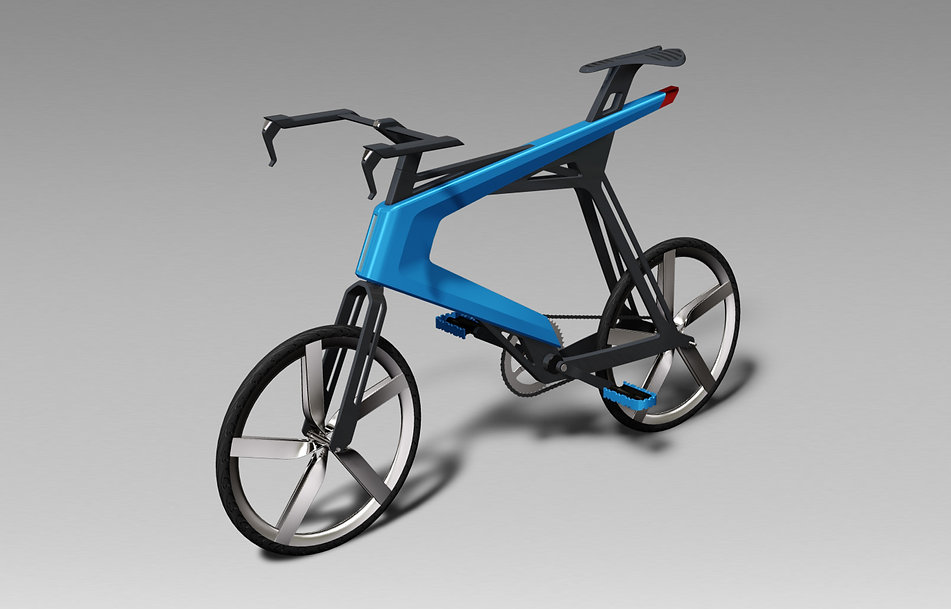A mini-velo concept with a V-shaped cover on a thin frame, lightweight optimization is achieved by applying the Voronoi Diagram theory, a computational geometry algorithm to the internal structure

Q1.  What is Voronoi diagram?

[A: Ph.D. Young-song Cho, Hanyang University]

Voronoi diagram (Voronoi diagram) is a mathematical methodology that divides space without gaps or overlaps. Given a two-dimensional plane, there are a variety of ways to divide a plane without gaps or overlaps. As shown in the picture below, the Korea traditional patchworks use only triangles to divide the plane (dividing the plane using one regular polygon is called mathematically regular tiling). Spain The bottom surface of the Sevilla Cathedral is divided into three planes (Archimedes) by triangles, squares, and hexagons (several regular polygons), and in the case of tiles of Yesil Camii (aka Green Temple) located in Bursa, Turkey, We are dividing the plane (Girhi Tiling). The exterior walls of the Water Cube in Beijing, where the Olympic Games were held in 2008, were created using Voronoi diagrams. In this way, Voronoi diagrams in 2D are another way of dividing planes without gaps or overlaps.(Regular tiling)

Sevilla Cathedral

(Archimedes tiling)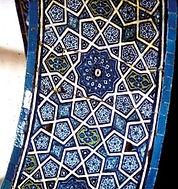​Yesil Cami

(Girih tiling)Beijing Water cube (Voronoi diagram)

Examples of various methods of dividing plane ​(Images : google)

The Voronoi diagram is named after the Ukrainian-born Russian mathematician George Voronoi (1868-1908) who studied this in earnest. The Voronoi diagram divides a plane into polygons that contain one point when there are points on the plane (point generator), but the point between any point inside the divided polygon and the point construct that the polygon contains Divides the distance so that it is closer to the other point constructors outside the polygon.

The Voronoi diagram is classified according to the kind of constructor such as 'Voronoi diagram of point' when the constructor is a point and 'Voronoi diagram of a circle' when it is a circle, and the area (Voronoi area) containing the point constructor in the 'Voronoi diagram of the point' In the 'Voronoi diagram of a circle', the boundaries of the Voronoi region of the original constructor are defined as curves (mathematically hyperbolic), whereas polygons are defined as polygons.

The Voronoi Diagram is classified according to the kind of constructor. When the constructor is a point, ‘Voronoi diagram of point' and when it is a circle, ‘Voronoi diagram of the circle' and the area (Voronoi area) containing the point constructor in the ‘Voronoi Diagram of the point' in the ‘Voronoi diagram of a circle',George Voronoi

Voronoi diagram of pointsVoronoi diagram of circle

George Voronoi and Voronoi diagram ​(Images : google)

Voronoi diagrams are easily found in nature. As shown in the picture below, the wings of a dragonfly, the epidermal pattern of a giraffe, the bottom of a lake divided by a drought, and the pillar-shaped joint of Jeju Island are examples of Voronoi diagrams that can be found in nature.Cracked lake bottomGiraffe patternsColumnar joint in Jeju

Voronoi diagram in nature ​(Images : google)

Q2. Can the same theory apply to three-dimensional space?

[A: Ph.D. Young-song Cho, Hanyang University]

Yes. The same theory applies in three dimensions(3D). In the case of the Voronoi diagram of points, the Voronoi region of the point constructor is a polygon in two dimensions, while the polygon is in three dimensions. Therefore, the Voronoi diagram of a three-dimensional point divides a three-dimensional space into a gap or a non-overlapping polyhedron. Similar to the Voronoi diagram of a circle in two dimensions, there is a Voronoi diagram of a sphere in three dimensions, where the Voronoi area of ​​a sphere constructor is defined as a surface (mathematically hyperbolic) with dots of the same distance between two adjacent sphere constructors.The Voronoi region of the point constructor in the Voronoi diagram of a three-dimensional pointThe Voronoi region of the sphere constructor (centered yellow sphere) in the Voronoi diagram of a three-dimensional point

Voronoi diagram in three-dimensional space (Image : Voronoi diagram research center, Hanyang University)

Q3. Which field is Voronoi diagram mainly used for?

[A: Ph.D. Young-song Cho, Hanyang University]

A Voronoi diagram is a method of dividing a space based on a constructor, which contains neighbor information between constructors and accessibility information with arbitrary points in space. Therefore, it can be applied to all problems that divide the space and analyze efficiently. For example, seismic stations should be deployed as soon as possible to detect seismic waves, and various types of public institutions, such as fire stations, government offices, and police stations, should be divided into jurisdictions for convenient access by people. Voronoi diagrams can play a key role. In recent years, Voronoi diagrams have also been used for facial recognition of people using cameras, and they are also used to analyze the structure of molecules such as proteins in order to develop new drugs. In addition, Voronoi diagrams are applied to the design.Optimal Disposition of a seismological observatory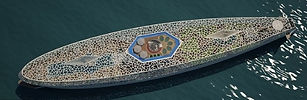Design

Examples of Voronoi diagram ​(Images : google)

Q4. Can Voronoi diagram theory be utilized in bicycle design?

[A: Sun-woong Hwang, Design manager at KLIO]

A bicycle is a tool that moves by manpower, except for an electric bicycle, so it is inevitably sensitive to weight. It is also important to ensure proper stiffness as people will continue to stress the frame continuously while driving. Both weight and stiffness are important, but there are many conflicting parts. For example, when light weight is made, there is a part where the rigidity is sacrificed, and when it is made with the importance of rigidity, the weight is sometimes increased. In some cases, both the weight and the stiffness are satisfied, but in this case, the price is often expensive.

Therefore, balancing the weight and rigidity is a key element of bicycle frame design. To analyze the stress (force) applied to the bicycle frame, stress on each part of the bicycle frame is different from stress on each part.

When these factors are reflected in the design of the bicycle frame, the parts that require a lot of stiffness become thicker, the parts that need less rigidity become thinner, and most bicycles become difficult to escape from this form.

However, using the Voronoi diagram makes it possible for the designer to add the Voronoi diagram more densely to the areas where the stiffness is needed, while maintaining the desired design, ensuring rigidity and lighter weight. As a result, design freedom can be enhanced, and other types of bicycle frame designs can be expected.The process of forming a lightweight structure using the Voronoi diagram is described.

(From left, KLIO : Sun-woong Hwang, KATECH : Jong-kwan Ra, Gwang-kyu Im, Do-hyun Kim, Hanyang University : Young-song Cho)

Q5. What are the specific stresses that often occur in bicycle frames?

[A: Sun-woong Hwang, Design manager at KLIO]

The stress applied to the bicycle frame can be seen as the force applied in every situation that occurs when a user rides on a bicycle. The power applied to all situations, such as pedaling, braking, etc., and the user's weight are constantly transmitted to the frame, and the forces applied in all of these situations are the stresses that the bike frame receives.Amount of stress on each part of the bicycle frame

Q6. What is the form most bikes can not escape from, and what does it mean to break out of it?

[A: Sun-woong Hwang, Design manager at KLIO]

The bike was completed in the late 1800s and has not changed much in its basic form so far. It has several meanings: first, it is a stable structure; second, it means that there is no change in bicycle production methods 200 years ago or now. Since the current bicycle frame, which is manpower-driven, is manufactured in a country where most labor costs are low, the quality varies according to who makes it, and it is difficult to deviate greatly from the standardized current shape for mass production. The standardized current bicycle has brought the monopoly of several companies, and most frame companies produce and sell only standard sizes.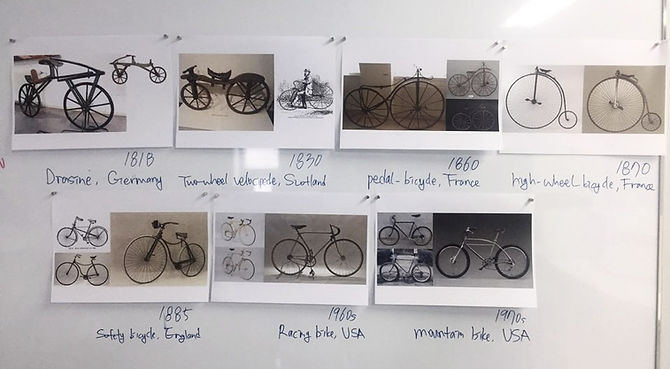History of Bicycle frame

This makes it very difficult for consumers who want a new type of bike or a frame that does not fit the standard size. The bicycle frame design combined with the Voronoi diagram is expected to enable differentiated frame design to meet the needs of users due to its high design freedom, which will create new demand different from the existing one.

Q7. What was the design of the proposed design concept (Voronoi Bike Concept) specifically?

[A: Sun-woong Hwang, Design manager at KLIO]

We made a sketch in the direction of escaping the existing form and made 3D data based on this sketch. The 3D data was analyzed based on the stress applied to each part of the bicycle frame and the physical properties of the frame (aluminum). Based on the analysis results, it is possible to maintain the rigidity through the optimization using the Voronoi diagram, Has been lightweight within.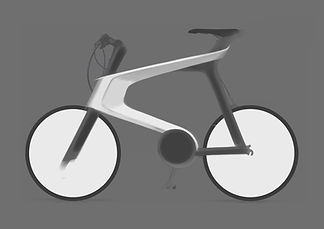Idea sketch (KLIO DESIGN)3D modeling (KLIO DESIGN)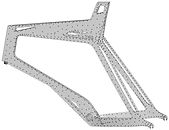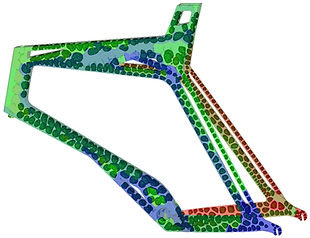The Voronoi diagram was applied to the frame design through the algorithm developed by Hanyang University.
After the input point (site) is generated at the point where the vertical load and the pedaling load act on the frame,
The Voronoi diagram empties the structure by generating cell structure.

(Voronoi diagram research center, Hanyang University)

Q8. What elements are needed to interpret the load on the bicycle frame?

[A: Gwang-kyu Im, KATECH Researcher]

Safety, function, durability, and accuracy are tested according to standards such as DIN EN 14764, DIN EN 14765, etc. The interpretation applied to this concept model is the vertical force and pedaling force. For more accurate analysis, the boundary conditions and the load conditions in the same conditions as the test conditions were constructed in the manner specified in EN 14764.Designers and engineers discussing the interpretation of the load on the frame and the data conversion process

Q9. How can I make it?

[A: Sun-woong Hwang, Design manager at KLIO]

If you apply Voronoi diagram, you will not be able to implement it because the Voronoi diagram distribution depends on the rigidity required for each part of the bicycle frame. So the way we choose is 3D printing. Because 3D printing has no major constraints on the shape, it is possible to create shapes that can not be realized with conventional production methods. In the future, we will be able to customize individual bicycles if we have only bicycle data so that we can produce bicycles through 3D printing.

​In order to check the product shape and assembly structure in actual size, we used a 3D printer to perform a mock-up. Specifically, in order to examine the design in a short time, 3D printing equipment that selectively injects the adhesive of plastic powder material (PMMA) is utilized. In order to confirm the Voronoi cell structure inside the frame, SLA (Stereolithography) equipment.3D printing for identification of Voronoi cell structure (3D Systems Korea)

1:1 size 3D Printing for design review

(DP-TECH)

Q10. What do you think is the right material to withstand load stress while maintaining design freedom?

[A: Gwang-kyu Im, KATECH Researcher]

I think that it is carbon which is made by piled up of multiple layers of carbon fiber and thermoforming. Carbon is very elastic, so it has a good ride and is very light in weight compared to other materials and has excellent strength. Due to the nature of the production, it is possible to adjust the thickness of each part to catch two rabbits of strength and light weight, and it is possible to make molding freely, and it is the best advantage that various shapes frame can be made, You can create frames with differentiated designs to suit your needs.

[A: Gwang-kyu Im, KATECH Researcher]

The disadvantage of carbon is the price. The price of the material can not be high because there is a lot of manual work in the process anyway.

Q11. What do you think is the future challenge for this concept to be implemented?

[A: Gwang-kyu Im, KATECH Researcher]

I think it is diversification of 3D printing materials. For example, bicycles must be secured by the person himself. It is said that it is possible to print using a metallic material through a 3D printer, but because the equipment is expensive, we think that printing material suitable for pursuing weight with strength enough to replace metal should be developed.

[A: Sun-woong Hwang, Design manager at KLIO]

I also think that 3D printing technology should be improved. Current 3D printing technology has some problems, but there are many technical aspects that need to be supplemented in order to use it for actual printing. I think that the problem of output speed, material problem, output quality is the most urgent problem. Even with improved surface quality in the case of layered stacking of materials, I think there is still a limit to making products that are under load, because the 'grain' of stacking can be vulnerable to impact and deformation. In addition, laser sintering can be superior in terms of quality and strength. In order to realize a "cell structure" such as a Voronoi diagram, the output method should be improved so that no material remains in each cell structure after the final output. If this part is complementary, I think we can create new demand in the market.

[Collaboration Consortium]
• Proposal and analysis of lightweight structural optimization methodology : KATECH
• Load study : PMRC Hongik University Personal mobility research center)
• Design : KLIO (KLIO DESIGN)
• Voronoi diagram based topology optimization : VDRC

(Hanyang Univ. Department of Mechanical Engineering Division of Computational Molecular center / Voronoi diagram research center)
• 3D Printing (Binder Jetting, PMMA) : DP TECH

• Voronoi structure sample 3D printing (SLA, Accura 25) : 3D systems Korea